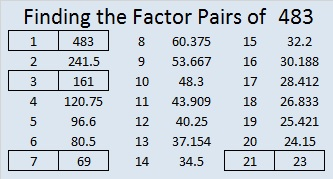# 483 and Level 3

4 + 8 = 12, a multiple of 3, so 483 can be evenly divided by 3. (It wasn’t necessary to add 4 + 8 + 3 because we already know 3 is divisible by 3.)

We can easily see that 483 is divisible by 7 if we apply the divisibility trick for 7. Separate the last number, 3, from the rest, double it, and subtract the double from the remaining numbers: 48 – (3 x 2) = 48 – 6 = 42, which is a multiple of 7. Thus 483 is divisible by 7.

Since 483 is 21 x 23, we can predict that 484 will be 22 x 22.Print the puzzles or type the solution on this excel file: 12 Factors 2015-05-04

—————————————————————————————————

• 483 is a composite number.
• Prime factorization: 483 = 3 x 7 x 23
• The exponents in the prime factorization are 1, 1, and 1. Adding one to each and multiplying we get (1 + 1)(1 + 1)(1 + 1) = 2 x 2 x 2 = 8. Therefore 483 has exactly 8 factors.
• Factors of 483: 1, 3, 7, 21, 23, 69, 161, 483
• Factor pairs: 483 = 1 x 483, 3 x 161, 7 x 69, or 21 x 23
• 483 has no square factors that allow its square root to be simplified. √483 ≈ 21.97726—————————————————————————————————